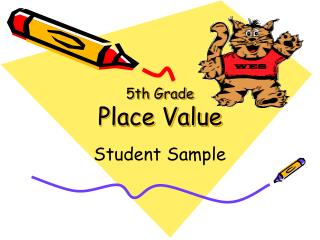DownloadDownload Presentation5th Grade Place Value

# 5th Grade Place Value

Download Presentation## 5th Grade Place Value

- - - - - - - - - - - - - - - - - - - - - - - - - - - E N D - - - - - - - - - - - - - - - - - - - - - - - - - - -
##### Presentation Transcript

1. 5th GradePlace Value Student Sample

2. Mississippi Framework Addressed Number Sense 5th Grade 1. Analyze relationships among numbers and the four basic operations, compute fluently, and make reasonable estimates.

3. Objectives I will be able to: • Write the standard, expanded, and word forms of whole numbers in the billions and identify the value of digits in whole numbers. • Compare and order whole numbers through billions. • Write decimals in standard form, word form, and expanded form through millionth. • Compare and order decimals through thousandths.

4. Vocabulary • Less than (<) – a symbol used to show numbers less than another • Equals (=) a symbol used to show numbers having the same value • Greater than (>) – a symbol used to show numbers greater than another

5. Vocabulary • Digit – The symbols used to show numbers: 0, 1, 2, 3, 4, 5, 6, 7, 8, and 9. • Standard Form – A common way of writing a number with commas separating groups of three digits starting from the right. • Word Form – A number written in words using place value. • Expanded Form – A way to write a number that shows the place value of each digit.

6. Place Value Chart

7. Standard Form 1,342,365.1427

8. Expanded Form 1,000,000+300,000+40,000+2,000+300+60+5+0.1000+0.0400+0.0020+0.0007

9. Word Form One Million, Three Hundred Forty-Two Thousand, Three Hundred Five and One Thousand Four Hundred Twenty-Seven Thousandths

10. How to compare numbers 1 2 3 Line up the numbers Compare at highest place value If numbers are the same go to next digit to the right 12,391 8,971 13,762 12,391 8,971 13,762 12,391 8,971 13,762

11. Write < > or = 53,931 < 54,931

12. Write < > or = 53,931 > 4,931

13. Write < > or = > 183,359 94,931

14. Write < > or = < 89,359 194,931

15. Write < > or = > 1,077 107

16. Write < > or = = 1,789 1,789

17. Write < > or = < 50,000 Five thousand

18. Write < > or = > 306 3 hundreds 6 tens

19. Write < > or = < 1,000+700+3 1,730

20. If you know how to compare numbers, you know how to put them in order Put these numbers in order from greatest to least 1,730 14 569 9,271 2 4 3 1

21. Put these numbers in order fromgreatest to least 35,872 36,872 569 9 2 1 3 4

22. Put these numbers in order fromleast to greatest 145,872 6,872 569 91 4 3 2 1

23. Put these numbers in order fromleast to greatest 5,872 5,832 5,991 910 3 2 4 1